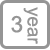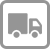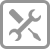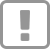20大吸力，方寸之间，油烟无踪

• 产品详情

--20大吸力，方寸之间，油烟无踪

BC805 顶吸式油烟机

^^^^^^^^^^^^^^^^^^^^^^^^^^^^^^^^^^^^^^^^^^^^^^^^^^^^^^^^^^^^^^^^^^^^^^^^^^^^^^^^^^^

20大吸力，方寸之间，油烟无踪

70MM缩进  退后一步，爱不磕碰

400pa大风压提高排风强度，强化排烟路径，即使遇到烹饪高峰和大风天气也能顺畅排烟不倒灌

3min延时关机设定一键定时功能，轻轻一按自动延时3分钟关机，省心又省力

53dB低噪音53dB的低噪音仅比夜间卧室高23dB,边烹饪边做其他事毫无压力，无论是听音乐，听课程，与人交流都能肆意享受

^^^^^^^^^^^^^^^^^^^^^^^^^^^^^^^^^^^^^^^^^^^^^^^^^^^^^^^^^^^^^^^^^^^^^^^^^^^^^^^^^^^

>

Professional security care for a better life

【享受服务】